Olympiad Test : High Order Thinking Skills - 2

# Olympiad Test : High Order Thinking Skills - 2

Test Description

## 20 Questions MCQ Test Maths Olympiad Class 6 | Olympiad Test : High Order Thinking Skills - 2

Olympiad Test : High Order Thinking Skills - 2 for Class 6 2022 is part of Maths Olympiad Class 6 preparation. The Olympiad Test : High Order Thinking Skills - 2 questions and answers have been prepared according to the Class 6 exam syllabus.The Olympiad Test : High Order Thinking Skills - 2 MCQs are made for Class 6 2022 Exam. Find important definitions, questions, notes, meanings, examples, exercises, MCQs and online tests for Olympiad Test : High Order Thinking Skills - 2 below.
Solutions of Olympiad Test : High Order Thinking Skills - 2 questions in English are available as part of our Maths Olympiad Class 6 for Class 6 & Olympiad Test : High Order Thinking Skills - 2 solutions in Hindi for Maths Olympiad Class 6 course. Download more important topics, notes, lectures and mock test series for Class 6 Exam by signing up for free. Attempt Olympiad Test : High Order Thinking Skills - 2 | 20 questions in 40 minutes | Mock test for Class 6 preparation | Free important questions MCQ to study Maths Olympiad Class 6 for Class 6 Exam | Download free PDF with solutions
 1 Crore+ students have signed up on EduRev. Have you?
Olympiad Test : High Order Thinking Skills - 2 - Question 1

### If 5 is subtracted from three times a number the result is 16. What is the number?

Detailed Solution for Olympiad Test : High Order Thinking Skills - 2 - Question 1

Let the number be x 3x – 5 = 16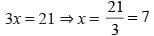Olympiad Test : High Order Thinking Skills - 2 - Question 2

### What is the share of B if Rs. 6900 is divided among A, B & C in the ratio 3 : 5 : 7?

Detailed Solution for Olympiad Test : High Order Thinking Skills - 2 - Question 2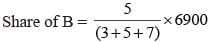= 2300

Olympiad Test : High Order Thinking Skills - 2 - Question 3

### The ratio of income to expenditure of Mohan is 7 : 5. What is the saving if the income is 14000?

Detailed Solution for Olympiad Test : High Order Thinking Skills - 2 - Question 3

Saving = 7 – 5 = 2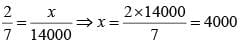Olympiad Test : High Order Thinking Skills - 2 - Question 4

What is the value of x if 16(3x – 5) – 10(4x – 8) = 40

Detailed Solution for Olympiad Test : High Order Thinking Skills - 2 - Question 4

16(3x – 5) – 10(4x – 8) = 40
48x – 80 – 40x + 80 = 40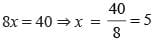Olympiad Test : High Order Thinking Skills - 2 - Question 5

The radius of a pencil is 6 mm and its length is 15 cm. What is the ratio of diameter of the pencil to the length of the pencil?

Detailed Solution for Olympiad Test : High Order Thinking Skills - 2 - Question 5

Diameter of the pencil = 6 × 2 = 12 mm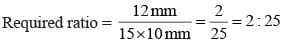Olympiad Test : High Order Thinking Skills - 2 - Question 6

If HCF and LCM of two numbers are 131 and 8253. One of the number is 917. What is the other?

Detailed Solution for Olympiad Test : High Order Thinking Skills - 2 - Question 6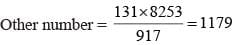Olympiad Test : High Order Thinking Skills - 2 - Question 7

What is the difference between place value and face value of 7 in 7063?

Detailed Solution for Olympiad Test : High Order Thinking Skills - 2 - Question 7

Difference = 7000 – 7 = 6993

Olympiad Test : High Order Thinking Skills - 2 - Question 8

What is the smallest 5-digit number divisible by 111?

Detailed Solution for Olympiad Test : High Order Thinking Skills - 2 - Question 8

Smallest five digit number = 10000
Required number = 10000 + (111 – 10) = 10101

Olympiad Test : High Order Thinking Skills - 2 - Question 9

What is the least number of 5-digits that is exactly divisible by 16, 18, 24 & 30?

Detailed Solution for Olympiad Test : High Order Thinking Skills - 2 - Question 9

LCM of 16, 18, 24, 30 = 1440
Smallest 5-digit number = 10000
Required number = 10000 + (1440 – 1360) = 10080

Olympiad Test : High Order Thinking Skills - 2 - Question 10

What is the value of 1 + 0.1 + 0.001?

Detailed Solution for Olympiad Test : High Order Thinking Skills - 2 - Question 10

1 + 0.1 + 0.01 + 0.001 = 1.111

Olympiad Test : High Order Thinking Skills - 2 - Question 11

0.213 ÷ 0.00213 = ?

Detailed Solution for Olympiad Test : High Order Thinking Skills - 2 - Question 11

0. 213 ÷ 0.00213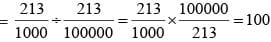Olympiad Test : High Order Thinking Skills - 2 - Question 12

What is the value of x if​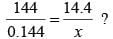Detailed Solution for Olympiad Test : High Order Thinking Skills - 2 - Question 12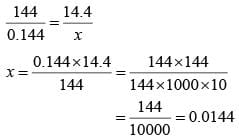Olympiad Test : High Order Thinking Skills - 2 - Question 13

What is the largest number of 4-digit exactly divisible by 12, 15, 18 & 27?

Detailed Solution for Olympiad Test : High Order Thinking Skills - 2 - Question 13

LCM of 12, 15, 18, 27 = 180
Largest number of 4-digit = 9999
Required number = 9999 – 99 = 9900

Olympiad Test : High Order Thinking Skills - 2 - Question 14

What is difference between two right angles and two straight angles?

Detailed Solution for Olympiad Test : High Order Thinking Skills - 2 - Question 14

Two right angles = 2 × 90° = 180°
Two straight angles = 2 × 180° = 360°
Difference = 360° – 180° = 180°

Olympiad Test : High Order Thinking Skills - 2 - Question 15

What is the value of P if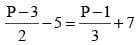Detailed Solution for Olympiad Test : High Order Thinking Skills - 2 - Question 15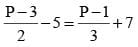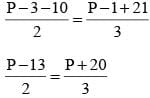3P – 39 = 2P + 40
3P – 2P = 40 + 39
P = 79

Olympiad Test : High Order Thinking Skills - 2 - Question 16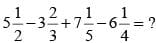Detailed Solution for Olympiad Test : High Order Thinking Skills - 2 - Question 16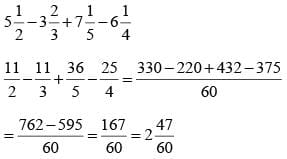Olympiad Test : High Order Thinking Skills - 2 - Question 17

What is the wrong number in the given sequence
1, 8, 27, 64, 124, 216, 343

Detailed Solution for Olympiad Test : High Order Thinking Skills - 2 - Question 17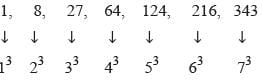Olympiad Test : High Order Thinking Skills - 2 - Question 18

Which is the largest fraction among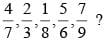Detailed Solution for Olympiad Test : High Order Thinking Skills - 2 - Question 18

LCM of 7, 3, 8, 6, 9 = 504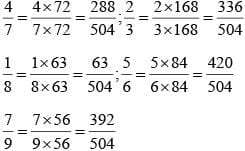5/6 is Largest

Olympiad Test : High Order Thinking Skills - 2 - Question 19

What will be the least number which when doubled will be exactly divisible by 12, 18, 21 & 30?

Detailed Solution for Olympiad Test : High Order Thinking Skills - 2 - Question 19

LCM of 12, 18, 21, 30 = 1260
Required number = 1260 ÷ 2 = 630

Olympiad Test : High Order Thinking Skills - 2 - Question 20

A car moves at a uniform speed of 65 km per hour. How much distance it will cover in 25 hours?

Detailed Solution for Olympiad Test : High Order Thinking Skills - 2 - Question 20

Distance = Speed × Time = 65× 25 = 1625 km

## Maths Olympiad Class 6

43 tests
 Use Code STAYHOME200 and get INR 200 additional OFF Use Coupon Code
Information about Olympiad Test : High Order Thinking Skills - 2 Page
In this test you can find the Exam questions for Olympiad Test : High Order Thinking Skills - 2 solved & explained in the simplest way possible. Besides giving Questions and answers for Olympiad Test : High Order Thinking Skills - 2, EduRev gives you an ample number of Online tests for practice

43 tests